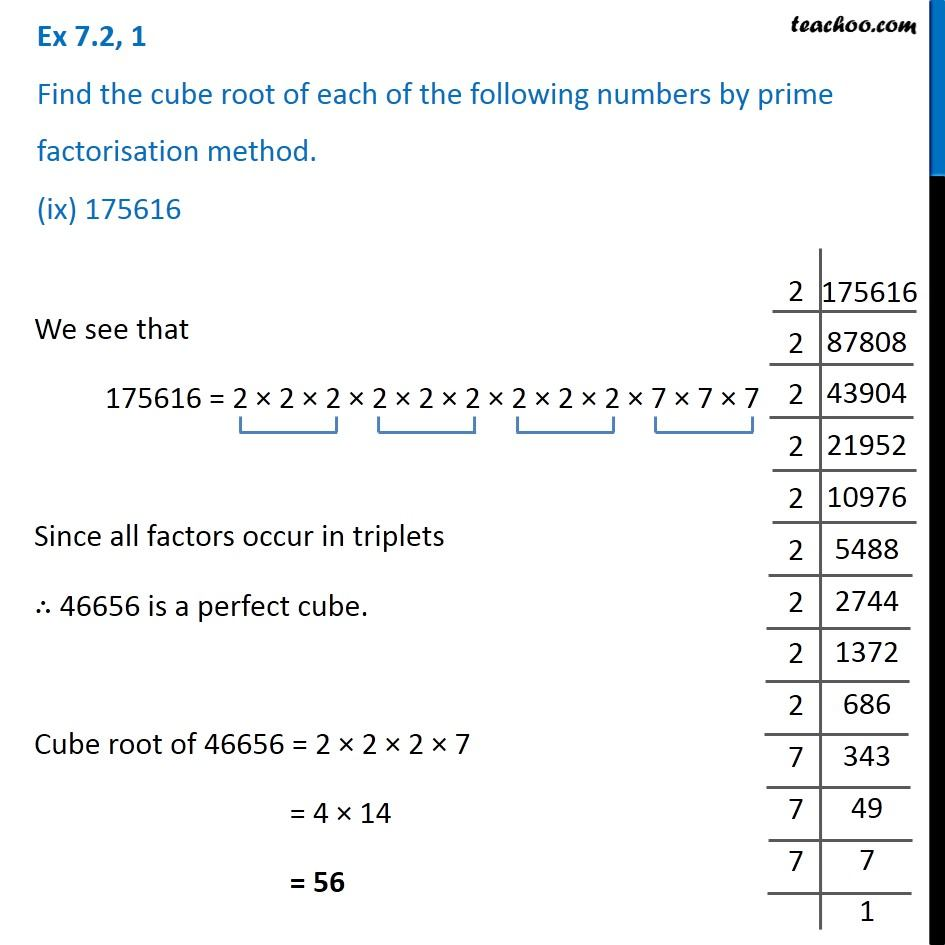Ex 7.2

Chapter 7 Class 8 Cubes and Cube Roots
Serial order wiseIntroducing your new favourite teacher - Teachoo Black, at only ₹83 per month

### Transcript

Ex 7.2, 1 Find the cube root of each of the following numbers by prime factorisation method. (ix) 175616We see that 175616 = 2 × 2 × 2 × 2 × 2 × 2 × 2 × 2 × 2 × 7 × 7 × 7 Since all factors occur in triplets ∴ 46656 is a perfect cube. Cube root of 46656 = 2 × 2 × 2 × 7 = 4 × 14 = 56